# 动态内存分配

## 动态内存分配的意义

### 简单点来说明

``````# include<stdio.h>
int main()
{

char array = "cyuyan";
char *string = "language";
printf("%s\n", *(&array));
printf("%s\n", string);
*(&array) = "language";
string = "cyuyan";
printf("%s\n", *(&array));
printf("%s\n", string);
return 0;
}
``````E0137表达式必须是可修改的左值 动态内存 8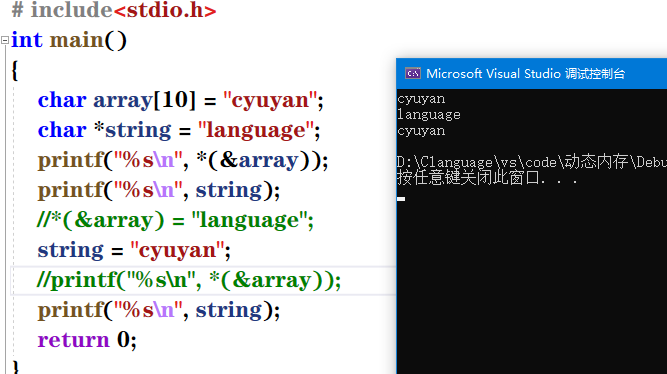## 动态内存分配的具体实现

### malloc函数的使用

• malloc函数的原型
``````void *malloc(unsigned int size)；
``````

• 具体的使用方法
``````# include<stdio.h>
# include<stdlib.h>
void InputNum()
{

int* array = (int*)malloc((sizeof(int)) * 11);
for (int i = 0; i <= 10; i++)
{

array[i] = i;
printf("%d ", array[i]);
}
}
int main()
{

InputNum();
return 0;
}
``````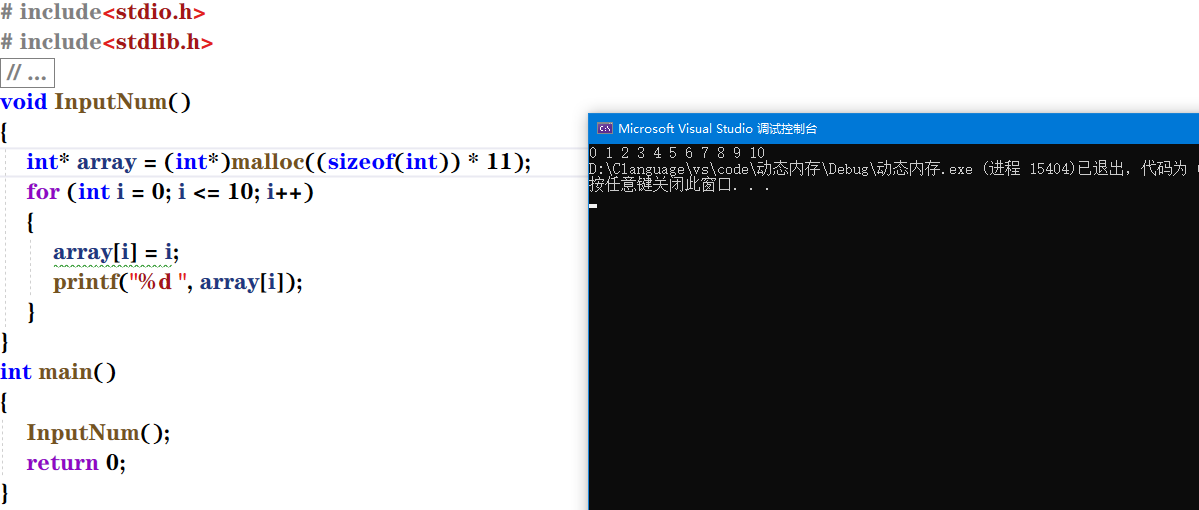``````# include<stdio.h>
# include<stdlib.h>
# include<errno.h>
void InputNum()
{

int* array = (int*)malloc((sizeof(int)) * 11);
if (array == NULL)
{

printf("%s", strerror(errno));
exit(EXIT_FAILURE);
}
for (int i = 0; i <= 10; i++)
{

array[i] = i;
printf("%d ", array[i]);
}
}
int main()
{

InputNum();
return 0;
}
``````

### calloc函数的使用

• calloc函数的原型
``````void *calloc(unsigned n,unsigned size);
``````

``````# include<stdio.h>
# include<stdlib.h>
# include<errno.h>
void InputNum()
{

int* array = (int*)malloc((sizeof(int)) * 11);
if (array == NULL)
{

printf("%s", strerror(errno));
exit(EXIT_FAILURE);
}
printf("array：");
for (int i = 0; i <= 10; i++)
{

array[i] = i;
printf("%d ", array[i]);
}
printf("\n");
//calloc函数
int* arr = calloc(11, sizeof(int));
printf("arr：");
if (arr == NULL)
{

printf("%s", strerror(errno));
exit(EXIT_FAILURE);
}
for (int j = 0; j <= 10; j++)
{

arr[j] = j;
printf("%d ", arr[j]);
}
}
int main()
{

InputNum();
return 0;
}
``````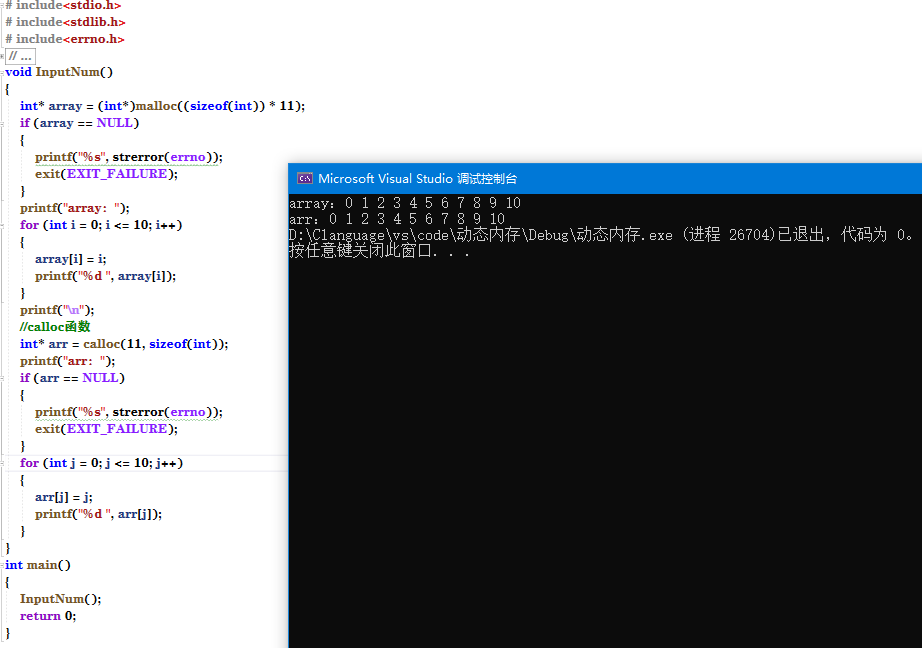``````# include<stdio.h>
# include<stdlib.h>
# include<errno.h>
void InputNum()
{

int* array = (int*)malloc((sizeof(int)) * 11);
if (array == NULL)
{

printf("%s", strerror(errno));
exit(EXIT_FAILURE);
}
printf("array：");
for (int i = 0; i <= 10; i++)
{

//array[i] = i;
printf("%d ", array[i]);
}
printf("\n");
//calloc函数
int* arr = calloc(11, sizeof(int));
printf("arr：");
if (arr == NULL)
{

printf("%s", strerror(errno));
exit(EXIT_FAILURE);
}
for (int j = 0; j <= 10; j++)
{

//arr[j] = j;
printf("%d ", arr[j]);
}
}
int main()
{

InputNum();
return 0;
}
``````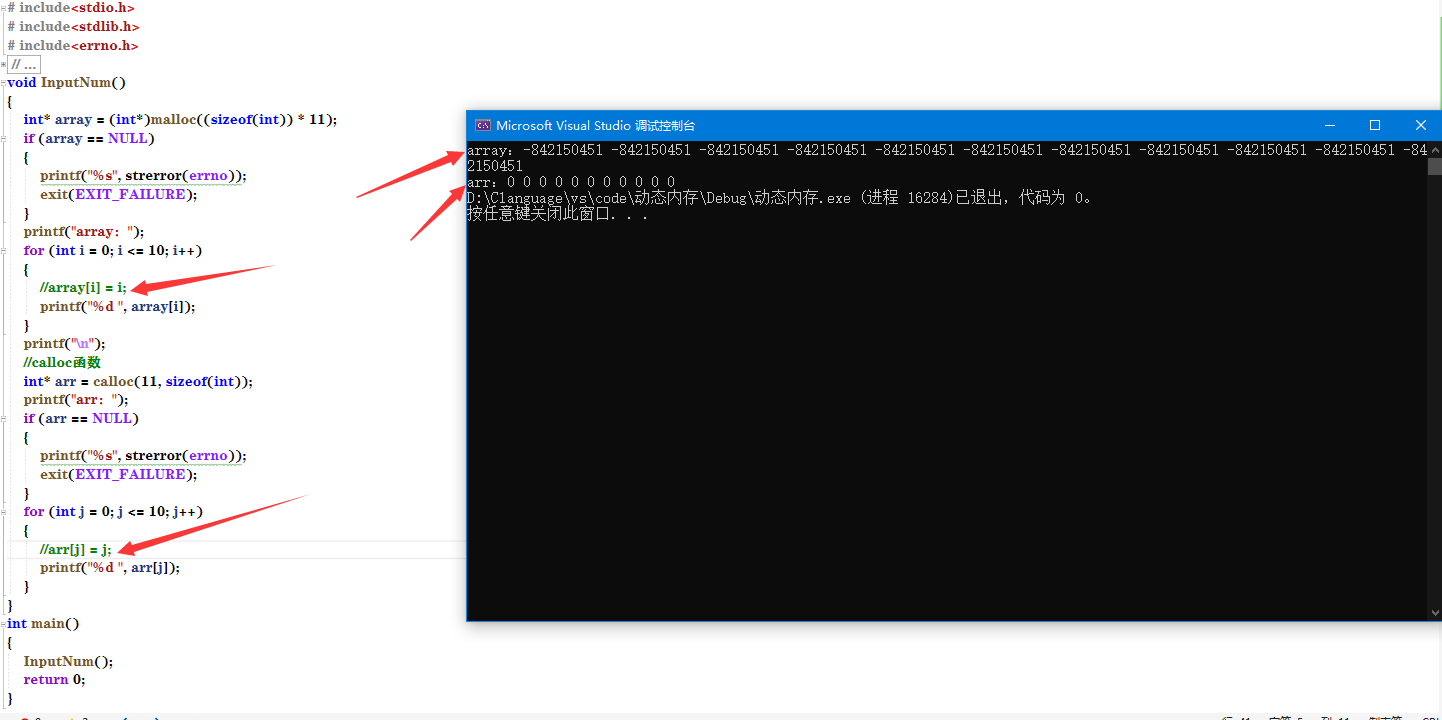### realloc函数的使用

• realloc函数的原型
``````void *realloc(void *mem_address, unsigned int newsize);
``````

``````# include<stdio.h>
# include<stdlib.h>
# include<errno.h>
void InputNum()
{

int* array = (int*)malloc((sizeof(int)) * 11);
if (array == NULL)
{

printf("%s", strerror(errno));
exit(EXIT_FAILURE);
}
printf("array：");
for (int i = 0; i <= 10; i++)
{

array[i] = i;
printf("%d ", array[i]);
}
printf("\n");
int* arr2 = (int*)realloc(array, sizeof(int) * 20);
for (int k = 0; k <= 19; k++)
{

printf("%d ", arr2[k]);
}
int main()
{

InputNum();
return 0;
}
``````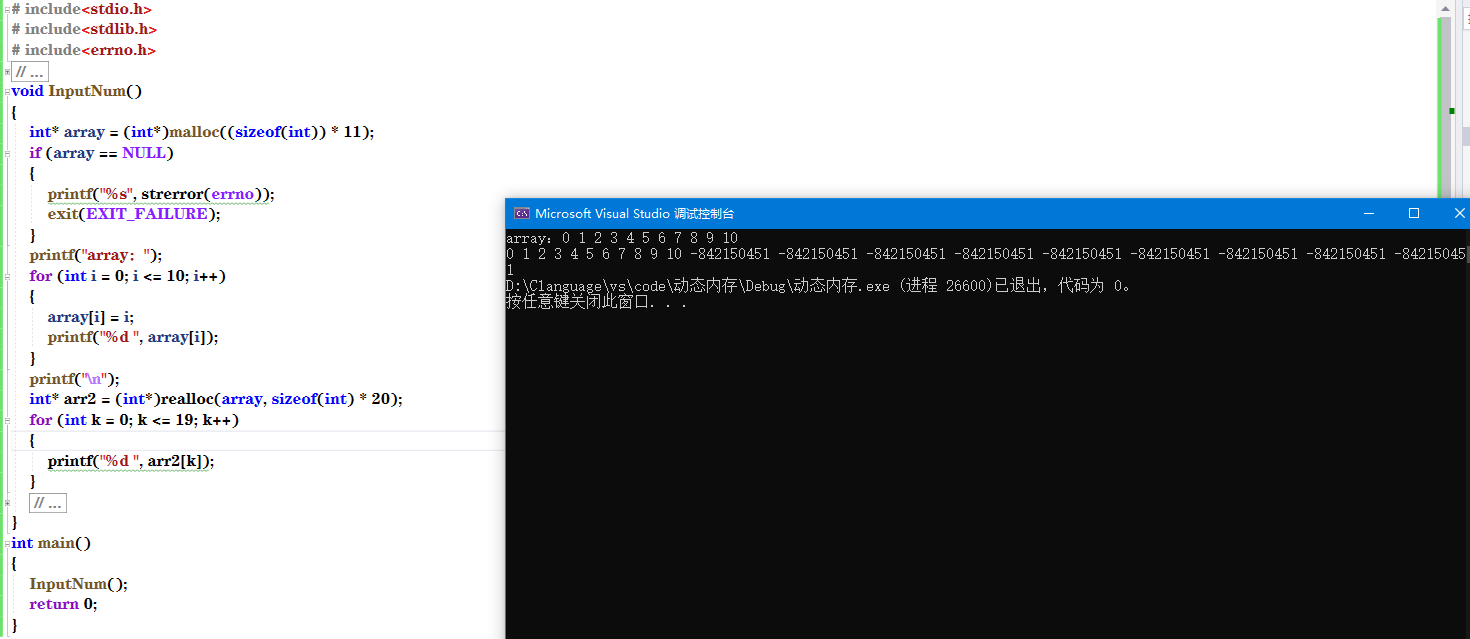## 动态内存分配中需要注意的问题

• 申请了的内存申请完毕后就要还回去
让我们来看看如果我们不还回去的话会出现什么问题
``````# include<stdio.h>
# include<stdlib.h>
# include<errno.h>
int main()
{

while (1)
{

int* p = (int*)malloc(sizeof(int) * 100);
}
return 0;
}
``````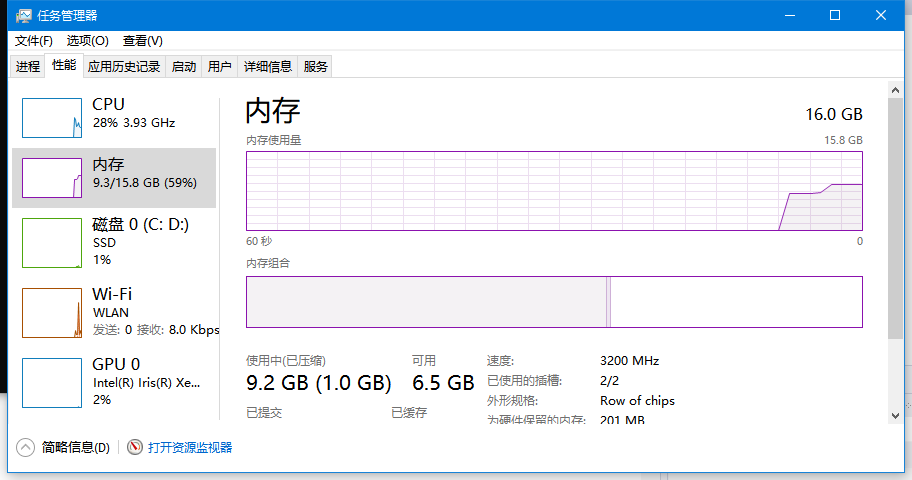### free函数的使用

``````# include<stdio.h>
# include<stdlib.h>
# include<errno.h>
int main()
{

while (1)
{

int* p = (int*)malloc(sizeof(int) * 100);
free(p);
p = NULL;
}
return 0;
}
``````

• 浏览(39)
• 浏览(29)
• 浏览(26)
• 浏览(24)
• 浏览(23)
• 浏览(22)
• 浏览(22)
• 浏览(22)
• 浏览(21)
• 浏览(19)